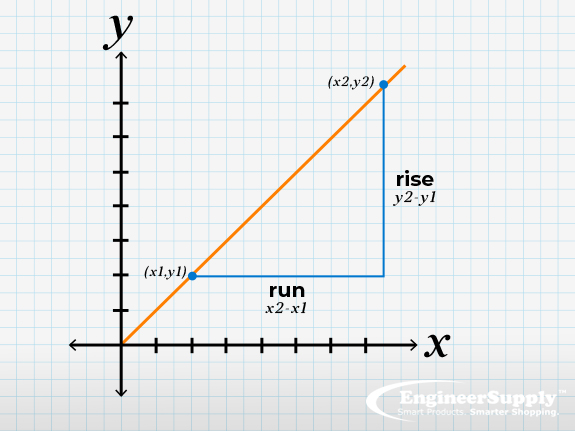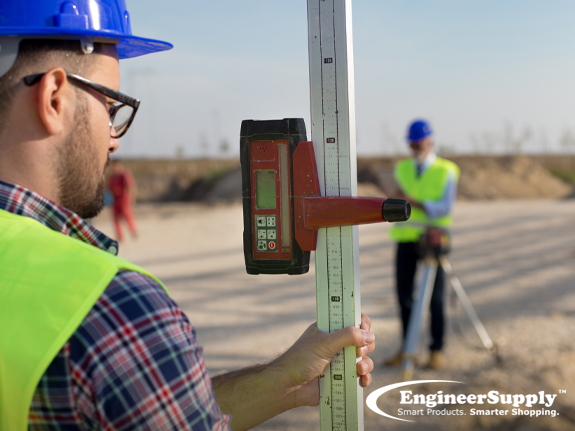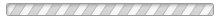# Understanding Slope and How it is MeasuredMaybe you're wanting to figure the slope of a drive way, a sanitary sewer pipe, a parking lot, a handicap accessible ramp, or maybe even the slope of a ditch. Also from time to time, you may hear people mention that something is at a percent slope and you wonder exactly what that means. If you take something like a ruler, pencil or stick and lay it on your desk, that's zero percent slope. Now, hold the object straight up. Straight up is 100 percent slope. Now you're getting the idea right? A 45-degree angle of the pencil results in a 50 percent slope. Lower it some more and you can easily get the idea of what 10% slope looks like, then 5 and even 2% slope.Many times slope plays a much more important role in design and in our lives in general than most people ever realize. The Americans with Disabilities Act (ADA) sets and enforces regulations with design criteria such as the slope of parking lots, sidewalks, and ramps. Sanitary sewer lines are usually installed at a 2% slope so that the water doesn't outrun the solids resulting in a clogged sewer line. Roads and highways are designed such that rainwater runs off yet is not sloped too steep making driving dangerous. There's literally thousands of examples where slope plays into our daily lives.

Slope is found often in the world of Civil Engineering, Design, Landscaping, and Construction. One must be able to fully understand what slope means and how it works. While we can talk and think about slope, we also need to know how to calculate and work with it.## Slope as a Percentage

How do you calculate the percentage grade of a slope? Slope can be calculated as a percentage which is calculated in much the same way as the gradient. Convert the rise and run to the same units and then divide the rise by the run. Multiply this number by 100 and you have the percentage slope. For instance, 3" rise divided by 36" run = .083 x 100 = an 8.3% slope.

Gaining a basic understanding of how to measure slope is important since you can then apply that knowledge to many other situations. Let's start by thinking about using wooden stakes, a good strong string, a string level, and a pocket tape measure to discover slope of the ground. The string level can be used to tie a string off in a level manner from stake to stake. Then using the tape measure to measure down from level the distance to a point on each end of the string. Then you take the distance from point to point along the string. Taking the difference in the two elevations you measured earlier, divide that figure by the length of the string you measured. From here you do the math to calculate slope as a percentage.

Now that you have this basic understanding of how to measure slope, you can then see how a transit or builders level could be used to measure down from level. The transit level sights a level line (invisible since its a sightline) to the grade rod (also known as a level rod) at one point along the distance you are measuring slope from. Then a reading is taken from level to the second spot. Once you know the distance from the two measured spots, and the distance from level on both spots, you can then do the math to calculate slope.

Other tools used for measuring slope would be laser levels, inclinometers, and slope meters.The field of construction can often be a demanding one. When you spend long hours at a site taking on an array of varied and difficult tasks, it is important to give yourself ample time to ensure you are utilizing the right tools for the job. Laser measuring tools are a cutting-edge development in the world of construction equipment that have made a serious impact on how easy it is to tackle tasks like measuring slope, determining elevation, and figuring out the grade of a particular piece of land you’re working on for a job.## Laser Measuring Tools Are Transforming Construction

In the past, the most effective way of measuring while working in the field of construction was by using a traditional measuring tape. While this is still an effective way of figuring out some measurements, the physical constraints of a tape measure can make it difficult to determine certain angles on terrain with unstable or uneven ground. A laser measurement device makes the process a lot easier by digitizing the process. All you need to do is point the device the direction you need to measure and look at the readout provided on the screen of the tool.

## The Advantages of Laser Measurement Devices

Perhaps the biggest advantage that comes with using laser measuring tools is the accuracy you can expect on the job. While conventional tape measures are useful, the overall precision of the device is limited by factors like human error and weather conditions. Measuring tools that use lasers are able to deliver accurate readings regardless of external factors that might otherwise result in incorrect data with traditional tools. Whether you predominantly work indoors or outside, taking advantage of this tech can completely transform the way you get through an average workday.

## What Do I Use To Measure a Hill’s Grade?

Measuring the grade of a hill is no small task. In order for you to get accurate measurements when figuring out the specific grade of a hill, you need to be able to rely on your tools. A laser measurement device can make all the difference in the accuracy of your readings.

## Can Laser Tools Measure Drainage Slopes?

Yes, you can easily use your tools to measure inclines for drainage. The most important part of this task is ensuring you have a secured tripod for your tool. Since drainage slopes are wet and slippery, you need a tripod base firmly planted in the ground to deliver accurate readings and limit the odds of a fall.## Can a Slope Be Found Easily on a Graph?

Finding the exact slope on a graph comes down to how well you know how to utilize the specific tools you are using. Before you take any laser tools out on the job, be sure to thoroughly read the manuals and play around with the features in order to feel confident in your ability to read the graph and execute commands.

## Is It Easy to Measure Elevation With Laser Tools?

As with slopes, it is entirely possible for you to use your laser measuring tools to figure out the elevation of a specific piece of land. As long as you lace the bottom of the leveling rod at the desired height, you should have no trouble getting a reading.

## Will My Tools Show Me Slope and Y Intercept?

Since understanding the exact numbers of a slope and Y-intercept can be crucial to the process, your laser tools will be able to provide you with this information. All you need to do is invest in the right tools, learn how to use them, and extract the most helpful data.
In order for you to deliver the best possible results during your next construction job, it can help to invest in the latest tools of the trade. Get a better feel for how these devices work by exploring the available selection at Engineer Supply and finding the right fit for your needs.

## How do I measure inclines?

You can determine how much the terrain rises between two points by subtracting the initial elevation point from the final elevation. If the elevation at the bottom of a hill is 200 feet and the elevation at the top of it is 1,000 feet, you would subtract 200 from 1,000 (which would give you 800). So in this situation, the incline between these two points is 800 feet.

## What is a 6% grade?

A 6% grade refers to the slope of a road, and the percent value means “per one hundred.” At a 6% grade, you increase your elevation by six units for every 100 units. So no matter which unit of measure is used, it’s important to keep them consistent at each point.A 6% grade refers to the slope of a road, and the percent value means “per one hundred.” At a 6% grade, you increase your elevation by six units for every 100 units. So no matter which unit of measure is used, it’s important to keep them consistent at each point.

## Is slope the same as gradient?

The two terms are similar to each other, but slope refers to a connection between two coordinate values. Gradient is like slope, except it refers to a single vector. This difference is important, because each part of the slope gradient indicates the rate of change with regard to that particular dimension.

## Why is it called "rise over run?"

If you want to know how to calculate slope, you find the ratio of the “vertical change” to the “horizontal change” between two points on a line. And sometimes it’s expressed as a quotient (called “rise over run”). The slope value will be the same number at every point on a straight line, which will depend on whether it’s moving upwards or downwards. This is the basic way on how to calculate slope.

## How do I find rise over run from two points?

Knowing how to calculate the slope of a line is an important skill for coordinate geometry, and it’s often used to draw lines on a graph or to determine its x and y intercepts. The slope of a line measures how steep it is between two points, which can be found by determining both the vertical and horizontal rates of change between two points.
If you're looking for a place to find quality survey equipment and engineering tools, be sure to look at what we have at Engineering Supply.

## Try Our Online Engineering Calculator

Please Wait...